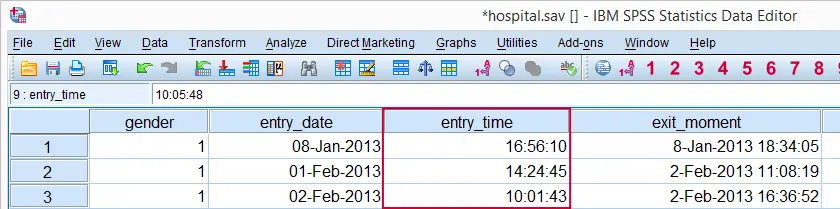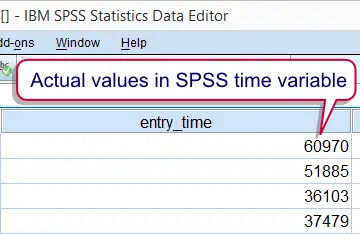SPSS TUTORIALS BASICS ANOVA REGRESSION FACTOR CORRELATION

# SPSS Time Variables Basics

## Introduction

Working efficiently with SPSS time variables is not hard if you understand some basics. This tutorial walks you through just those. We recommend you follow along by downloading and opening hospital.sav.## SPSS Time Variables - What Are They?

SPSS time variables are variables that hold time intervals in numbers of seconds. Although the actual time values are just simple numbers, they are usually displayed as hours, minutes and seconds. Don't let this appearance confuse you; it's the underlying numbers of seconds that time calculations act upon.
Note that SPSS time variables are numeric variables: all of SPSS' numeric functions can be used on them in the exact same way as you would on other numeric variables. However, we often use SPSS time functions for calculations on time variables.

## SPSS Common Time Formats

Time values are numbers of seconds but we usually display them as hours, minutes and seconds. We can do so by setting their format to one of SPSS' time formats. The table below shows the most common ones.

Variable TypeFormat familyFormat (example)Shown as
NumericTimeTime516:56
NumericTimeTime816:56:10

## SPSS Time Variables - Example

We'll now focus on entry_time in our data. We'll take a look at its actual values (numbers of seconds) in data view; running the following line of syntax will show them: formats entry_time(f1).Note that this doesn't change the values in any way; they're merely displayed differently. We can show them as normal time values again by running formats entry_time(time8). Knowing that time values are really just numbers of seconds makes it pretty easy to work with them. For instance, we can set all time values one hour ahead by simply adding 3600 (seconds in one hour) to them by running the syntax below.

## SPSS Time Variable Syntax Example

*1. Add 3600 seconds (= 1 hour) to all time values.

compute entry_time = entry_time + 3600.

*2. Sort cases on entry_time descendingly.

sort cases by entry_time(d).

Looking at the result, we notice something strange: the first couple of values exceed 24 hours. That is pretty odd in this particular case. However, it generally makes sense when we understand the nature of SPSS time values, which we'll discuss next.## SPSS Time Values

Keep in mind that SPSS time values contain time intervals. These often indicate clock times as intervals between midnight and a given time point. However, this is not necessarily the case.
For example, the duration of a hotel stay (difference between exit moment and arrival moment) will typically be entered as a time variable in SPSS. This time interval probably doesn't start at midnight and will often exceed 24 hours.
In short, SPSS time variables hold time spans that may or may not indicate clock times. SPSS time values may also exceed 24 hours because they don't contain a day or month component.

# Tell us what you think!

*Required field. Your comment will show up after approval from a moderator.

# THIS TUTORIAL HAS 1 COMMENT:

•